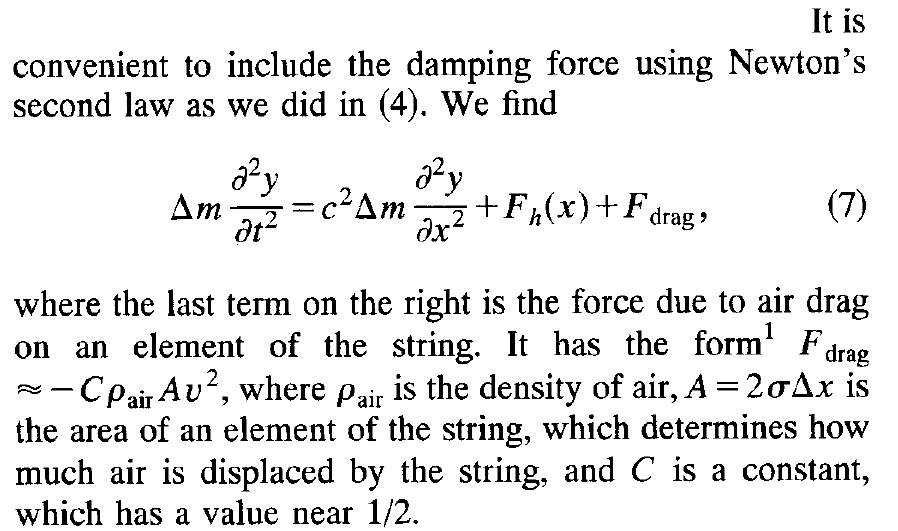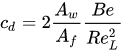# How do I read this equation for air friction/drag on an object?

• mikejm
In summary, the author is describing how to calculate the drag coefficient for a string, and defines area in terms of standard deviation. He also mentions that drag coefficients are velocity-dependent, and that a characteristic width of the string can be used to approximate area.f

#### mikejm

I am trying to understand an excerpt from an article describing the vibrations of a string (eg. guitar/piano) which reads as follows:This is basically the wave equation with Δm representing a small piece of mass from an interval of the string and two forces added to the right side.

He defines the drag from air friction as F = -C*A*v^2. I can accept this. However, he then defines area with A = 2 * σ * Δx. I'm not sure of what σ means here.

Δx would be expected to represent the length of the string fragment corresponding to Δm. But what is σ? How does this give you area of the string? I know volume of string segment would be V = π * r^2 * Δx. Cross sectional area would be A = π * r^2. If you tried to imagine it as a flat object like a billboard you could say A = 2 * r * Δx. So is that what he's doing? Is σ radius?

I've never seen it used that way and searching "σ radius" gives no results suggesting it's commonplace. He doesn't define sigma anywhere in the article, so I presume you're just supposed to know.

Is this likely the correct interpretation and is this normal notation for radius?

Thanks

It should not be the radius, because the normal equation for air drag includes ##v^2/2##.

https://www.grc.nasa.gov/www/k-12/airplane/drageq.html

https://en.m.wikipedia.org/wiki/Drag_coefficient

Another way of defining reference area that made sense to me for drag of a string would be taking half of the surface area of the string fragment, since half is pushing against the air at any given time.

This would be:

A = (π d Δx)/2

Though obviously some of the area is pushing more than others. Ie. The central area of the string would have the most force and the edges would induce less per unit of area.

Could he then mean σ in terms of standard deviation? Ie. Somehow trying to represent some percent of the area that would be representative?

From the NASA citation, from @Lnewqban,
In practice, drag coefficients are reported based on a wide variety of object areas. In the report, the aerodynamicist must specify the area used; when using the data, the reader may have to convert the drag coefficient using the ratio of the areas.
If the author did not explicitly define the area to be used for a determined drag coefficient C , then there is a bit of slop in his/her article, hence your inquiry.

A common choice is related to projected area, ( billboard ) exposed and moving relative to the air mass, such as you stated - 2 r Δx, where the σ would (could ) be the radius. But really, who would know except the author.

Though obviously some of the area is pushing more than others. Ie. The central area of the string would have the most force and the edges would induce less per unit of area.
Is the drag coefficient C represented spatially and temporally as as a function of location on the string element, and perhaps also time ( ie something like C( Θ, t ) in polar coordinates ) in this article? Which obviously doesn't make much sense, since the drag coefficient is that defined for the whole body, ( or parts of a body with little influence from other parts ) used for the ease of simpler computation.
For a simple shape such as a cylinder ( the guitar string ) these drag coefficients can be then tabulated.

Also,
as a blanket statement, that may or may not be true for any general shape.citing the Reynold's number, and the Bejan number, which makes the drag coefficient not as a constant, but velocity dependent.

I would guess he is simply referring to characteristic width of the string, i.e. the diameter. This means the area A is just the projected or cross section area of the string, which is quite normal to use in air drag calculations.

Edit: 256bits beat me to it

Thanks guys. As per the NASA link then the most correct formula would be:

D = Coeff * airdensity * Area * 0.5 * V^2

I will use a billboard style approximation of area = diameter * length.

Appreciate all the clarification. That should work well enough.

This is really just for approximation of the phenomenon and since it's scaled by a somewhat arbitrary coefficient that should be close enough.

Thanks again.

•Lnewqban